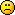Discussion about math, puzzles, games and fun.   Useful symbols: ÷ × ½ √ ∞ ≠ ≤ ≥ ≈ ⇒ ± ∈ Δ θ ∴ ∑ ∫  π  -¹ ² ³ °

You are not logged in.

## #1 Help Me ! » help with group theory please. » 2013-05-01 14:01:54

limpfisch
Replies: 1

let a,b be members of a group (G,*). Show that if (a*b) inverse = (a inverse)*(b inverse) then a*b = b*a

## #2 Help Me ! » Householder transformation? » 2012-09-12 12:05:37

limpfisch
Replies: 1

Perform a householder transformation followed by a plane rotation to trasform the following matrix A into an upper triangular matrix R.

A= 4 3 0
4 3 6
2 6 5

This is not covered in my textbook## #3 Help Me ! » more linear algebra help please? » 2012-09-12 11:56:58

limpfisch
Replies: 0

Let S = {(1234),(4321)}
and W the subspace of R4 generated by S.

Find an orthogonal basis of the orthogonal complement W^ of W
Find the shortest distance from u+(12-21) to W^

Obviously the vectors of S are meant to be written as one colum instead of 4.
Thanks.

## #4 Help Me ! » Linear algebra help » 2012-09-12 11:39:28

limpfisch
Replies: 0

Show that if A and B represent reflections in R2 or R3, then AB represents a rotation.

I can invision this but i'm not sure how to show it.

## #5 Help Me ! » Show that C is also a basis of V » 2012-08-16 04:59:41

limpfisch
Replies: 2

Let B = {u1,u2} be a basis of a vector space V
Show that C = {v1 = 2u1 - 3u2, v2 = 2u1 + 2u2} is also a basis of V.

Also, what is the change of basis matrix from B to C?

Thanks very much for any help you give me.

## #6 Help Me ! » Change of basis of matricies. » 2012-08-16 00:13:01

limpfisch
Replies: 1

Let B= {(2 -1),(3 -1)
Let C= {(1 2),(2 3)

Find the change of basis matrix from C to B.

I've been sick the last week so haven't attended lectures and have an assignment due. The notes for this course are terrible and do not help me with this so please explain how to do this thanks.

## #7 Help Me ! » question to do with double integrals over rectangles » 2012-05-20 14:41:42

limpfisch
Replies: 1

Let R1 denote the rectangle [0, 5] × [4, 4], R2 the rectangle [0, 5] × [0, 4], and R3 the
rectangle [5, 0] × [4, 0]. Suppose that ∫∫R2 f (x, y)dA = 10 , that ∫∫R3 f (x, y)dA = 24
and that f (−x, y) = − f (x, y) for all (x, y). Evaluate ∫∫R1 f (x, y)dA

really not sure on the approach here.

## #8 Help Me ! » Find the unit tangent vector T(t) » 2012-05-09 19:29:13

limpfisch
Replies: 1

r(t) = ((4cost)^3, (4sint)^3)  for -pi<=pi

i tried just finding T(t) by using r'(t)/|r'(t)| but i keep getting a giant mess which is no good given i have to find the normal vector and curvature later. Please help.

## #9 Help Me ! » Please help me with this problem » 2012-05-09 16:27:27

limpfisch
Replies: 1

On a certain mountain, the elevation z above a point (x, y) in a horizontal xy-plane
at sea level is z = 2500 - x^2/10 - y^2/5   metres.  The positive x-axis points east; the
positive y-axis points north. Suppose that a climber is at the point (15, 10, 2457.5)

if the climber moves southease, what rate will the climber begin to descend at?

i calculated the gradients in the zx direction and in the zy direction, but i'm not sure what the continuation is.

## #10 Re: Help Me ! » Which spring requires more material to make? » 2012-05-09 14:24:23

It's ok i got it after about 2 hours of thinking. I used the formula for a helix and then found the absolute value of it's derivative and went from there. Turns out the 2nd spring does use a little more spring.....i think.

## #11 Help Me ! » Which spring requires more material to make? » 2012-05-08 17:06:33

limpfisch
Replies: 8

Which spring requires more material to make it, one of radius 5 cm and height 4 cm
that makes three complete turns or one of radius 3 cm and height 4 cm that makes
five complete turns?

i thought i did it right but logically my answer can't be.